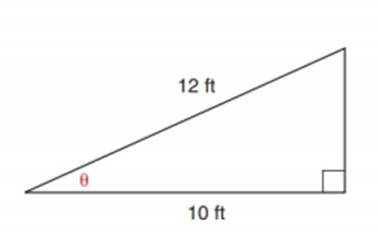Chapter 11.2, Problem 29EElementary Geometry For College St...

7th Edition
Alexander + 2 others
ISBN: 9781337614085

Solutions

Chapter
SectionElementary Geometry For College St...

7th Edition
Alexander + 2 others
ISBN: 9781337614085
Textbook Problem

In Exercise 29 to 37, angle measures should be given to the nearest degree; distances should be given to the nearest tenth of a unit.In adding a garage onto his house, Gene wants to use a sloped 12-ft roof to cover an expanse that is 10 ft. wide. Find the measure of angle θTo determine

To find:

To find the measure of angle θ by using the following condition,

“Gene wants to use a sloped 12-ft roof to cover an expanse that is 10 ft. wide”

Explanation

Consider the following figure,

General formula for cosine ratio and sin ratio is given below,

From the given figure the value 12 ft

Still sussing out bartleby?

Check out a sample textbook solution.

See a sample solution

The Solution to Your Study Problems

Bartleby provides explanations to thousands of textbook problems written by our experts, many with advanced degrees!

Get Started

Find more solutions based on key concepts